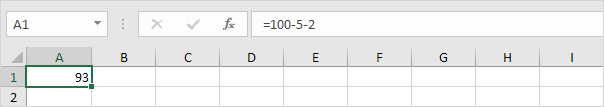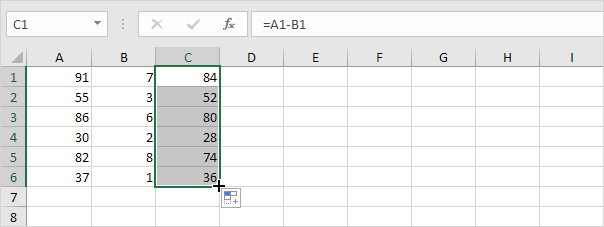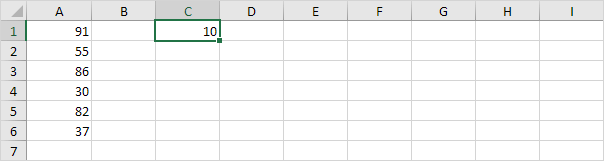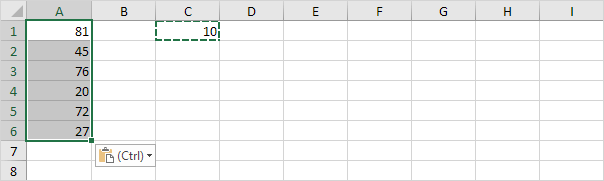# Subtract

There’s no SUBTRACT function in Excel. However, there are several ways to subtract numbers in Excel. Are you ready to improve your Excel skills?

1. For example, the formula below subtracts numbers in a cell. Simply use the minus sign (-) as the subtraction operator. Don’t forget, always start a formula with an equal sign (=).2. The formula below subtracts the value in cell A2 and the value in cell A3 from the value in cell A1.3. As you can imagine, this formula can get quite long. Simply use the SUM function to shorten your formula. For example, the formula below subtracts the values in the range A2:A9 from the value in cell A1.Take a look at the screenshot below. To subtract the numbers in column B from the numbers in column A, execute the following steps.

4a. First, subtract the value in cell B1 from the value in cell A1.4b. Next, select cell C1, click on the lower right corner of cell C1 and drag it down to cell C6.Take a look at the screenshot below. To subtract a number from a range of cells, execute the following steps.

5a. First, subtract the value in cell A8 from the value in cell A1. Fix the reference to cell A8 by placing a \$ symbol in front of the column letter and row number (\$A\$8).5b. Next, select cell B1, click on the lower right corner of cell B1 and drag it down to cell B6.Explanation: when we drag the formula down, the absolute reference (\$A\$8) stays the same, while the relative reference (A1) changes to A2, A3, A4, etc.

If you’re not a formula hero, use Paste Special to subtract in Excel without using formulas!

6. For example, select cell C1.7. Right click, and then click Copy (or press CTRL + c).

8. Select the range A1:A6.

9. Right click, and then click Paste Special.

10. Click Subtract.11. Click OK.Note: to subtract numbers in one column from numbers in another column, at step 6, simply select a range instead of a cell.